Courses

# Chemical Equilibrium ( Chapter Test - Medical)

## 20 Questions MCQ Test | Chemical Equilibrium ( Chapter Test - Medical)

Description
This mock test of Chemical Equilibrium ( Chapter Test - Medical) for Class 12 helps you for every Class 12 entrance exam. This contains 20 Multiple Choice Questions for Class 12 Chemical Equilibrium ( Chapter Test - Medical) (mcq) to study with solutions a complete question bank. The solved questions answers in this Chemical Equilibrium ( Chapter Test - Medical) quiz give you a good mix of easy questions and tough questions. Class 12 students definitely take this Chemical Equilibrium ( Chapter Test - Medical) exercise for a better result in the exam. You can find other Chemical Equilibrium ( Chapter Test - Medical) extra questions, long questions & short questions for Class 12 on EduRev as well by searching above.
QUESTION: 1

Solution:
QUESTION: 2

Solution:
QUESTION: 3

### In the reaction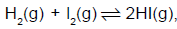value of the equilibrium constant depends on

Solution:
QUESTION: 4

The conditions which favour the manufacture of ammonia by the reaction (Le-Chatelier’s principle)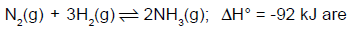Solution:
QUESTION: 5

1 mole of H2 and 2 moles of I2 are taken initially in a 5 litre vessel. If the number of moles of H2 at equilibrium is 0.2, the number of moles of I2 and HI, respectively at equilibrium would be

Solution:
QUESTION: 6

Consider the reaction  2H2 + O22H2O.

Which of the following correctly states the expression for the equilibrium constant for this reaction?

Solution:
QUESTION: 7

1.2 moles each of pure acetic acid and 1.2 moles of pure ethanol are subjected to esterification. At
equilibrium 0.4 moles of ester were found to have formed. The equilibrium constant for the reaction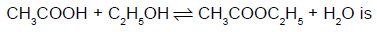Solution:
QUESTION: 8

At a rather high temperature the Kp values are computed as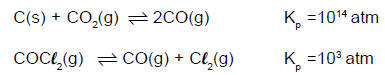What is the Kp for the reaction ?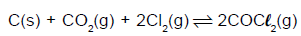Solution:
QUESTION: 9

Kc for a reaction A + 2B ⇔ D, is 0.64. What should be the concentration of B when it is in equilibrium with
equimolar quantities of A and D ?

Solution:
QUESTION: 10

In which of the following, Kp is not greater than Kc?

Solution:
QUESTION: 11

Given the equation,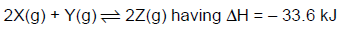Which combination of pressure and temperature should give the best yield of Z ?

Solution:
QUESTION: 12

The equilibrium constant Kp for the reaction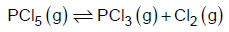is 16. If the volume of the container is reduced to one half of its original volume,the value of Kp for the reaction at the same temperature will be

Solution:
QUESTION: 13

The equilibrium constant for the given reaction is 100.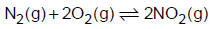What is the equilibrium constant for the reaction given below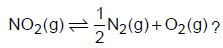Solution:
QUESTION: 14

For the reaction,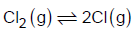the formation of Cl(g) is favoured at

Solution:
QUESTION: 15

In the system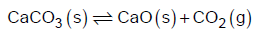doubling the mass of CaO will cause the equilibrium concentration of CO2 to

Solution:
QUESTION: 16

In equilibrium constant expression, concentration of products is taken in/on the

Solution:
QUESTION: 17

Given the equilibrium reaction,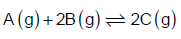This reaction is studied by starting with equal amounts of A and B in a constant-volume vessel. Which of the following expressions will hold good ?

Solution:
QUESTION: 18

In a system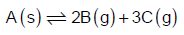if the equilibrium concentration of B increases to 8 times, it will cause the equilibrium concentration of C to change to

Solution:
QUESTION: 19

For the heterogeneous equilibrium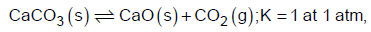the temperature is given by

Solution:
QUESTION: 20

Given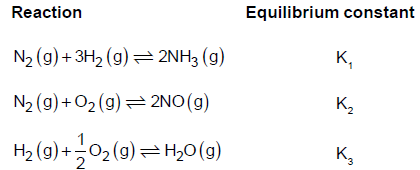The equilibrium constant K4 for the following reaction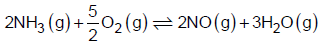may be given as

Solution: